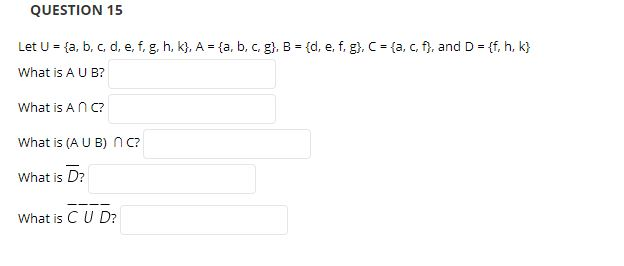# QUESTION 15Let U {a, b, c, d, e, f. g. h, k), A {a , b, c. g), B (d, e. f. g), C ta, c, f, and D ff, h. k)What is A U B?What is A n CWhat is (A U B) n c?What is D?What is C U D?

Questionhelp_outlineImage TranscriptioncloseQUESTION 15 Let U {a, b, c, d, e, f. g. h, k), A {a , b, c. g), B (d, e. f. g), C ta, c, f, and D ff, h. k) What is A U B? What is A n C What is (A U B) n c? What is D? What is C U D? fullscreen
check_circle

Step 1

Hello there! there are more than three sub parts in the question. According to our policies  cannot answer more than three sub parts, Do find solutions for first three parts. If you have any doubts in remaining parts kindly request as a new question.

Given sets A, B, C, D as shown below
Union of two sets is denoted by symbol U and it includes all the elements in both the sets.
Intersection of two sets is denoted by symbol ∩  and it includes only the elements which are common in both the sets.

Step 2

The set A U B is calculated as shown below
A U B ={ a,b,c,d,e,f,g }

Step 3

The set A ∩ C is calculated as shown b...

### Want to see the full answer?

See Solution

#### Want to see this answer and more?

Solutions are written by subject experts who are available 24/7. Questions are typically answered within 1 hour.*

See Solution
*Response times may vary by subject and question.
Tagged in

### Basic Probability